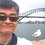# $1^\infty$-Limit Cases

This is to prove that if $\displaystyle \lim_{x \to \infty} f(x) = 1$ and $\displaystyle \lim_{x \to \infty} g(x) = \infty$, then $\displaystyle \lim_{x \to \infty} f(x)^{g(x)} = e^{\lim_{x \to \infty} g(x)(f(x) - 1)}$.

\begin{aligned} \lim_{x \to \infty} f(x)^{g(x)} & = \lim_{x \to \infty} \left(1+f(x)-1\right)^{g(x)} \\ & = \lim_{x \to \infty} \left(1+\frac 1{\frac 1{f(x)-1}} \right)^{g(x)\left(\frac {f(x)-1}{f(x)-1}\right)} \\ & = \lim_{x \to \infty} \left[\left(1+\frac 1{\frac 1{f(x)-1}} \right)^\frac 1{f(x)-1}\right]^{g(x)(f(x)-1)} \\ & = \lim_{x \to \infty} e^{g(x)(f(x)-1)} \\ & = e^{\lim_{x \to \infty} g(x)(f(x)-1)} \end{aligned}Note by Chew-Seong Cheong
1 year, 10 months ago

This discussion board is a place to discuss our Daily Challenges and the math and science related to those challenges. Explanations are more than just a solution — they should explain the steps and thinking strategies that you used to obtain the solution. Comments should further the discussion of math and science.

When posting on Brilliant:

• Use the emojis to react to an explanation, whether you're congratulating a job well done , or just really confused .
• Ask specific questions about the challenge or the steps in somebody's explanation. Well-posed questions can add a lot to the discussion, but posting "I don't understand!" doesn't help anyone.
• Try to contribute something new to the discussion, whether it is an extension, generalization or other idea related to the challenge.

MarkdownAppears as
*italics* or _italics_ italics
**bold** or __bold__ bold
- bulleted- list
• bulleted
• list
1. numbered2. list
1. numbered
2. list
Note: you must add a full line of space before and after lists for them to show up correctly
paragraph 1paragraph 2

paragraph 1

paragraph 2

[example link](https://brilliant.org)example link
> This is a quote
This is a quote
    # I indented these lines
# 4 spaces, and now they show
# up as a code block.

print "hello world"
# I indented these lines
# 4 spaces, and now they show
# up as a code block.

print "hello world"
MathAppears as
Remember to wrap math in $$ ... $$ or $ ... $ to ensure proper formatting.
2 \times 3 $2 \times 3$
2^{34} $2^{34}$
a_{i-1} $a_{i-1}$
\frac{2}{3} $\frac{2}{3}$
\sqrt{2} $\sqrt{2}$
\sum_{i=1}^3 $\sum_{i=1}^3$
\sin \theta $\sin \theta$
\boxed{123} $\boxed{123}$

Sort by:

Hello Sir! How are you? Remember me? I got admitted to an IIT this year!

- 1 year, 6 months ago

Yes, how are you?

- 1 year, 6 months ago

I am fine sir, How are you?

- 1 year, 5 months ago

- 1 year, 5 months ago

IIT bhu right

- 1 year, 1 month ago

Yeah! Do i know you?

- 1 year, 1 month ago

No,bhaiya i was on your group on quora

- 1 year, 1 month ago

Okay bro nice! Which class you are in?

- 1 year, 1 month ago

Bhaiya,I am in 10 th class.Can you give me some tips regarding boards and prmo.....and should i learn computer languages or just focus on boards???Please reply.

- 1 year, 1 month ago

Boards mein kuch ni rkha h bro. Thoda padhlio obviously, kyuki aas pados wale puchte hai. Baaki computer language seekh le,agar accha lgta hai, continue with that. It will help a lot in future.

- 1 year, 1 month ago

Aur bhaiya prmo ke liye........

- 1 year, 1 month ago

you contact me through quora, im inactive here kriti.

- 11 months, 2 weeks ago

Ok bhaiya!

- 11 months, 2 weeks ago

Zuhair is cool :)

- 9 months, 1 week ago

Bravo! You are awesome, Chew-Seong Cheong. I have seen your solutions all over Brilliant! I could use your help with a couple of mathematical mysteries if you are willing. I will start with one concerning (x'(t))^2+(y'(t))^2=1. (I did the work for this by myself, so there could be a mistake.) Please respond when you have time, and I will convey the trouble I am having with this topic. Again, if you are willing...

- 6 months, 2 weeks ago